# Tracking¶

This tutorial introduces the pvlib.tracking module. This module currently only contains one function, tracking.singleaxis, but we hope to add dual axis tracking support in the future.

The tracking.singleaxis function is a port of the PVLIB MATLAB file pvl_singleaxis.m. The algorithm is based on Lorenzo et al, Tracking and back-tracking, Prog. in Photovoltaics: Research and Applications, 19, 747-753 (2011). Most of the Python and MATLAB algorithms are identical except for name changes to conform to the PEP8 Python style guide. There are few spots, noteably in the calculation of surface_azimuth, that our implementation differs from the MATLAB implementation.

This tutorial requires pvlib >= 0.6.0.

This tutorial was written by

• Will Holmgren (@wholmgren), University of Arizona. March, 2015, July 2015, March 2016, April 2016, August 2018.
• Some of the text is based on the comments in pvl_singleaxis.m, presumably written by the PVLIB_MATLAB team at Sandia National Laboratory.

## Setup¶

Standard scientific Python imports.

In :
# plotting modules
%matplotlib inline
import matplotlib.pyplot as plt

# built in python modules
import datetime

import numpy as np
import pandas as pd

In :
import pvlib
from pvlib.tools import cosd, sind
from pvlib.location import Location


Make some pvlib Location objects. These are the standard inputs to the solar position calculator.

In :
tus = Location(32.2, -111, 'US/Arizona', 700, 'Tucson')
print(tus)
johannesburg = Location(-26.2044, 28.0456, 'Africa/Johannesburg', 1753, 'Johannesburg')
print(johannesburg)

Location:
name: Tucson
latitude: 32.2
longitude: -111
altitude: 700
tz: US/Arizona
Location:
name: Johannesburg
latitude: -26.2044
longitude: 28.0456
altitude: 1753
tz: Africa/Johannesburg


Calculate solar position at those locations. To start, we'll choose times near an equinox. Later, we'll test against times near a solstice.

In :
times = pd.date_range(start=datetime.datetime(2014,3,23), end=datetime.datetime(2014,3,24), freq='5Min')

ephem_tus = pvlib.solarposition.get_solarposition(times.tz_localize(tus.tz), tus.latitude, tus.longitude)
ephem_joh = pvlib.solarposition.get_solarposition(times.tz_localize(johannesburg.tz),
johannesburg.latitude, johannesburg.longitude)
ephemout = ephem_tus # default for notebook


## Single axis tracker algorithm¶

### Inputs¶

First, define the input parameters. From the tracking.singleaxis docstring...

apparent_zenith : Series
Solar apparent zenith angles in decimal degrees.

azimuth : Series
Solar azimuth angles in decimal degrees.

latitude : float
A value denoting which hempisphere the tracker is
in. The exact latitude is NOT required, any positive number denotes
the northern hemisphere, any negative number denotes the southern
hemisphere, a value of 0 is assumed to be northern hemisphere.

axis_tilt : float
The tilt of the axis of rotation
(i.e, the y-axis defined by axis_azimuth) with respect to horizontal,
in decimal degrees.

axis_azimuth : float
A value denoting the compass direction along which
the axis of rotation lies, in decimal degrees.

max_angle : float
A value denoting the maximum rotation angle, in
decimal degrees, of the one-axis tracker from its horizontal position
(horizontal if axis_tilt = 0).
A max_angle of 90 degrees allows the tracker to rotate to a vertical
position to point the panel towards a horizon.
max_angle of 180 degrees allows for full rotation.

backtrack : bool
Controls whether the tracker has the
capability to "backtrack" to avoid row-to-row shading.
False denotes no backtrack capability.
True denotes backtrack capability.

gcr : float
A value denoting the ground coverage ratio of a tracker
system which utilizes backtracking; i.e. the ratio between the PV
array surface area to total ground area. A tracker system with modules 2
meters wide, centered on the tracking axis, with 6 meters between the
tracking axes has a gcr of 2/6=0.333. If gcr is not provided, a gcr
of 2/7 is default. gcr must be <=1.
In :
azimuth = ephemout['azimuth']
apparent_azimuth = ephemout['azimuth']
apparent_zenith = ephemout['apparent_zenith']
axis_tilt = 10
axis_azimuth = 170
latitude = 32
max_angle = 65
backtrack = True
gcr = 2.0/7.0

times = azimuth.index


### Transform to south facing coordinate system¶

The reference that this algorithm is based on used an Earth coordinate system where y points south. So, we first transform our solar position vector to this new coordiante system.

In :
az = apparent_azimuth - 180
apparent_elevation = 90 - apparent_zenith
x = cosd(apparent_elevation) * sind(az)
y = cosd(apparent_elevation) * cosd(az)
z = sind(apparent_elevation)

earth_coords = pd.DataFrame({'x':x,'y':y,'z':z})

earth_coords.plot()
plt.title('sun position in Earth coordinate system')

Out:
Text(0.5,1,'sun position in Earth coordinate system')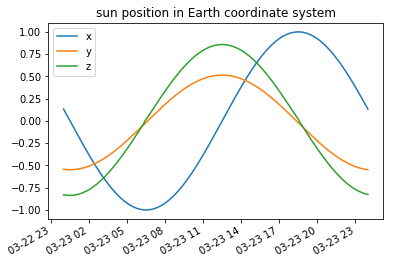### Transform to panel coordinate system¶

Transform solar vector to panel coordinate system. For North-South oriented trackers parallel to the ground, the only difference is the sign of the x component. The x components are the same if axis_azimuth=180 and opposite if axis_azimuth=0.

In :
axis_azimuth_south = axis_azimuth - 180

print('cos(axis_azimuth_south)={}, sin(axis_azimuth_south)={}'
.format(cosd(axis_azimuth_south), sind(axis_azimuth_south)))
print('cos(axis_tilt)={}, sin(axis_tilt)={}'
.format(cosd(axis_tilt), sind(axis_tilt)))

xp = x*cosd(axis_azimuth_south) - y*sind(axis_azimuth_south);
yp = (x*cosd(axis_tilt)*sind(axis_azimuth_south) +
y*cosd(axis_tilt)*cosd(axis_azimuth_south) -
z*sind(axis_tilt))
zp = (x*sind(axis_tilt)*sind(axis_azimuth_south) +
y*sind(axis_tilt)*cosd(axis_azimuth_south) +
z*cosd(axis_tilt))

panel_coords = pd.DataFrame({'x':xp,'y':yp,'z':zp})

panel_coords.plot()
plt.title('sun position in panel coordinate system')

cos(axis_azimuth_south)=0.984807753012208, sin(axis_azimuth_south)=-0.17364817766693033
cos(axis_tilt)=0.984807753012208, sin(axis_tilt)=0.17364817766693033

Out:
Text(0.5,1,'sun position in panel coordinate system')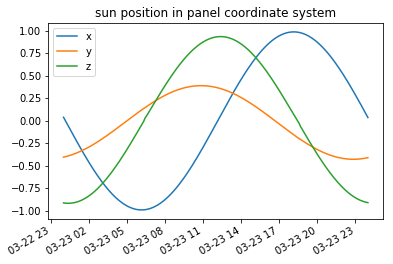### Tracking angle¶

The ideal tracking angle wid is the rotation to place the sun position vector (xp, yp, zp) in the (y, z) plane; i.e. normal to the panel and containing the axis of rotation. wid = 0 indicates that the panel is horizontal. Here, our convention is that a clockwise rotation is positive, to view rotation angles in the same frame of reference as azimuth. For example, for a system with tracking axis oriented south, a rotation toward the east is negative, and a rotation to the west is positive.

We use arctan2, but PVLIB MATLAB uses arctan. Here prove that we get the same result.

In :
# Calculate angle from x-y plane to projection of sun vector onto x-z plane
# and then obtain wid by translating tmp to convention for rotation angles.
wid = pd.Series(90 - np.degrees(np.arctan2(zp, xp)), index=times)

# filter for sun above panel horizon
wid[zp <= 0] = np.nan

wid.plot(label='tracking angle')
ephemout['apparent_elevation'].plot(label='apparent elevation')
plt.legend()
plt.title('Ideal tracking angle without backtracking')

Out:
Text(0.5,1,'Ideal tracking angle without backtracking')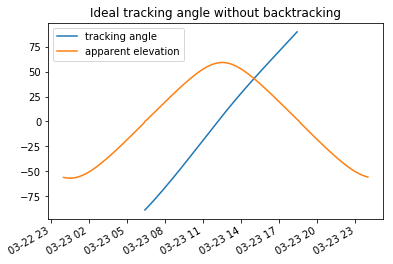arctan version

In :
tmp = np.degrees(np.arctan(zp/xp))  # angle from x-y plane to projection of sun vector onto x-z plane

# Obtain wid by translating tmp to convention for rotation angles.
# Have to account for which quadrant of the x-z plane in which the sun
# vector lies.  Complete solution here but probably not necessary to
# consider QIII and QIV.
wid = pd.Series(index=times)
wid[(xp>=0) & (zp>=0)] =  90 - tmp[(xp>=0) & (zp>=0)];  # QI
wid[(xp<0)  & (zp>=0)] = -90 - tmp[(xp<0)  & (zp>=0)];  # QII
wid[(xp<0)  & (zp<0)]  = -90 - tmp[(xp<0)  & (zp<0)];   # QIII
wid[(xp>=0) & (zp<0)]  =  90 - tmp[(xp>=0) & (zp<0)];   # QIV

# filter for sun above panel horizon
wid[zp <= 0] = np.nan

wid.plot(label='tracking angle')
ephemout['apparent_elevation'].plot(label='apparent elevation')
plt.legend()
plt.title('Ideal tracking angle without backtracking')

Out:
Text(0.5,1,'Ideal tracking angle without backtracking')### Backtracking¶

Account for backtracking; modified from  to account for rotation angle convention being used here.

In :
if backtrack:
axes_distance = 1/gcr
temp = np.minimum(axes_distance*cosd(wid), 1)

# backtrack angle
# (always positive b/c acosd returns values between 0 and 180)
wc = np.degrees(np.arccos(temp))

v = wid < 0
widc = pd.Series(index=times)
widc[~v] = wid[~v] - wc[~v]; # Eq 4 applied when wid in QI
widc[v] = wid[v] + wc[v];    # Eq 4 applied when wid in QIV
else:
widc = wid

widc.plot(label='tracking angle')
#pyephemout['apparent_elevation'].plot(label='apparent elevation')
plt.legend(loc=2)
plt.title('Ideal tracking angle with backtracking')

Out:
Text(0.5,1,'Ideal tracking angle with backtracking')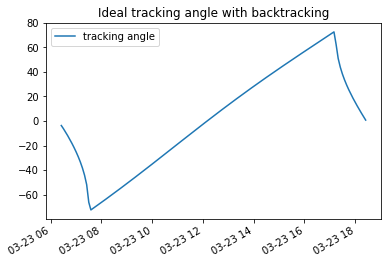Compare tracking angle with and without backtracking.

In :
tracking_angles = pd.DataFrame({'with backtracking':widc,'without backtracking':wid})
tracking_angles.plot()
#pyephemout['apparent_elevation'].plot(label='apparent elevation')
plt.legend()

Out:
<matplotlib.legend.Legend at 0x111ac8f98>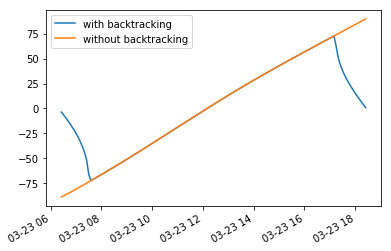### Max angle¶

Apply angle restriction.

In :
tracker_theta = widc.copy()
tracker_theta[tracker_theta > max_angle] = max_angle
tracker_theta[tracker_theta < -max_angle] = -max_angle

tracking_angles['with restriction'] = tracker_theta
tracking_angles.plot()

Out:
<matplotlib.axes._subplots.AxesSubplot at 0x111924e10>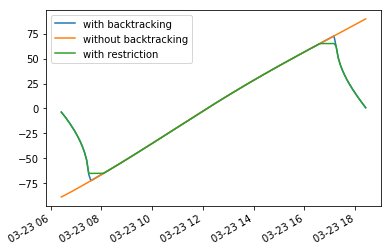### Calculate panel normal¶

Calculate panel normal vector in panel x, y, z coordinates. y-axis is axis of tracker rotation. tracker_theta is a compass angle (clockwise is positive) rather than a trigonometric angle.

In :
panel_norm = np.array([sind(tracker_theta),
tracker_theta*0,
cosd(tracker_theta)])

panel_norm_df = pd.DataFrame(panel_norm.T, columns=('x','y','z'), index=times)
panel_norm_df.plot()
plt.title('panel normal vector components in panel coordinate system')
plt.legend()

Out:
<matplotlib.legend.Legend at 0x111c49e10>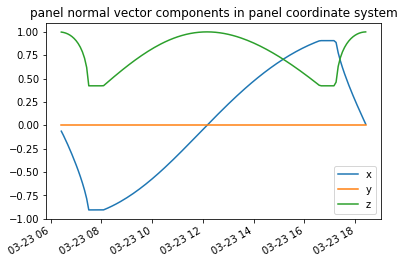sun position in vector format in panel-oriented x, y, z coordinates. We've already seen this above, but it's good to look at it again after calculating the tracker normal vector.

In :
sun_vec = np.array([xp, yp, zp])

panel_coords = pd.DataFrame(sun_vec.T, columns=('x','y','z'), index=times)

panel_coords.plot()
plt.title('sun position in panel coordinate system')

Out:
Text(0.5,1,'sun position in panel coordinate system')### AOI¶

Calculate angle-of-incidence on panel

In :
aoi = np.degrees(np.arccos(np.abs(np.sum(sun_vec*panel_norm, axis=0))))
aoi = pd.Series(aoi, index=times)

aoi.plot()
plt.title('angle of incidence')

Out:
Text(0.5,1,'angle of incidence')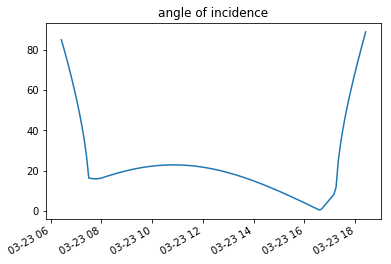The power produced by the tracker will be primarily determined by the cosine of the angle of incidence.

In :
cosd(aoi).plot()

Out:
<matplotlib.axes._subplots.AxesSubplot at 0x111fde2b0>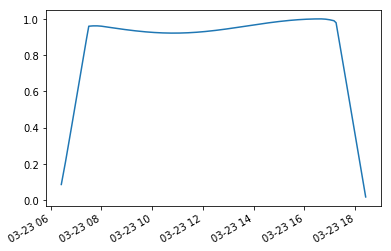### Surface tilt and azimuth¶

Calculate panel tilt surface_tilt and azimuth surface_azimuth in a coordinate system where the panel tilt is the angle from horizontal, and the panel azimuth is the compass angle (clockwise from north) to the projection of the panel's normal to the earth's surface. These outputs are provided for convenience and comparison with other PV software which use these angle conventions.

Project normal vector to earth surface. First rotate about x-axis by angle -axis_tilt so that y-axis is also parallel to earth surface, then project.

In :
# Calculate standard rotation matrix
print('cos(axis_azimuth_south)={}, sin(axis_azimuth_south)={}'
.format(cosd(axis_azimuth_south), sind(axis_azimuth_south)))
print('cos(axis_tilt)={}, sin(axis_tilt)={}'
.format(cosd(axis_tilt), sind(axis_tilt)))

rot_x = np.array([[1, 0, 0],
[0, cosd(-axis_tilt), -sind(-axis_tilt)],
[0, sind(-axis_tilt), cosd(-axis_tilt)]])

# panel_norm_earth contains the normal vector expressed in earth-surface coordinates
# (z normal to surface, y aligned with tracker axis parallel to earth)
panel_norm_earth = np.dot(rot_x, panel_norm).T

# projection to plane tangent to earth surface,
# in earth surface coordinates
projected_normal = np.array([panel_norm_earth[:,0], panel_norm_earth[:,1], panel_norm_earth[:,2]*0]).T

# calculate magnitudes
panel_norm_earth_mag = np.sqrt(np.nansum(panel_norm_earth**2, axis=1))
projected_normal_mag = np.sqrt(np.nansum(projected_normal**2, axis=1))
#print('panel_norm_earth_mag={}, projected_normal_mag={}'.format(panel_norm_earth_mag, projected_normal_mag))

projected_normal = (projected_normal.T / projected_normal_mag).T

panel_norm_earth_df = pd.DataFrame(panel_norm_earth, columns=('x','y','z'), index=times)
panel_norm_earth_df.plot()
plt.title('panel normal vector components in Earth coordinate system')

projected_normal_df = pd.DataFrame(projected_normal, columns=('x','y','z'), index=times)
projected_normal_df.plot()
plt.title('panel normal vector projected to surface in Earth coordinate system')

cos(axis_azimuth_south)=0.984807753012208, sin(axis_azimuth_south)=-0.17364817766693033
cos(axis_tilt)=0.984807753012208, sin(axis_tilt)=0.17364817766693033

Out:
Text(0.5,1,'panel normal vector projected to surface in Earth coordinate system')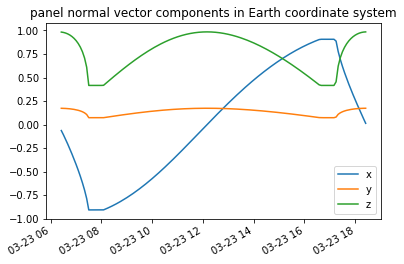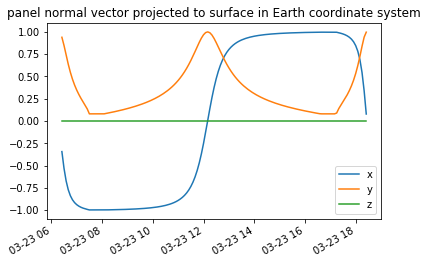#### Surface azimuth¶

Calculate surface_azimuth. This takes a few steps. We need to take the arctan, rotate from the panel system to the south-facing Earth system and then rotate the Earth system to a north-facing Earth system. We use the arctan2 function, but PVLIB MATLAB uses arctan.

In :
# calculation of surface_azimuth
# 1. Find the angle.
surface_azimuth = pd.Series(np.degrees(np.arctan2(projected_normal[:,1], projected_normal[:,0])), index=times)
surface_azimuth.plot(label='orig')

# 2. Rotate 0 reference from panel's x axis to it's y axis and
#    then back to North.
surface_azimuth = 90 - surface_azimuth + axis_azimuth

# 3. Map azimuth into [0,360) domain.
surface_azimuth[surface_azimuth<0] += 360
surface_azimuth[surface_azimuth>=360] -= 360
surface_azimuth.plot(label='compass angle north')

plt.legend()

Out:
<matplotlib.legend.Legend at 0x112168b38>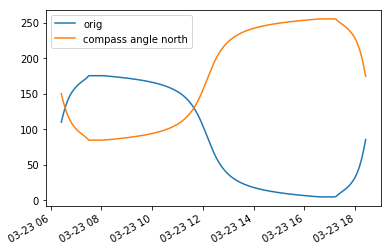arctan version

In :
# calculation of surface_azimuth
# 1. Find the angle.
surface_azimuth = pd.Series(np.degrees(np.arctan(projected_normal[:,1]/projected_normal[:,0])), index=times)
surface_azimuth.plot(label='orig')

# 2. Clean up atan when x-coord or y-coord is zero
surface_azimuth[(projected_normal[:,0]==0) & (projected_normal[:,1]>0)] =  90
surface_azimuth[(projected_normal[:,0]==0) & (projected_normal[:,1]<0)] =  -90
surface_azimuth[(projected_normal[:,1]==0) & (projected_normal[:,0]>0)] =  0
surface_azimuth[(projected_normal[:,1]==0) & (projected_normal[:,0]<0)] = 180
surface_azimuth.plot(label='x or y 0 corrected')

# 3. Correct atan for QII and QIII
surface_azimuth[(projected_normal[:,0]<0) & (projected_normal[:,1]>0)] += 180 # QII
surface_azimuth[(projected_normal[:,0]<0) & (projected_normal[:,1]<0)] += 180 # QIII
surface_azimuth.plot(label='q2, q3 corrected')

# From PVLIB MATLAB...
# at this point surface_azimuth contains angles between -90 and +270,
# where 0 is along the positive x-axis,
# the y-axis is in the direction of the tracker azimuth,
# and positive angles are rotations from the positive x axis towards
# the positive y-axis.
# (clockwise rotation from 0 along the positive y-axis)
#    surface_azimuth[surface_azimuth<=90] = 90 - surface_azimuth[surface_azimuth<=90]
#    surface_azimuth[surface_azimuth>90] = 450 - surface_azimuth[surface_azimuth>90]

# finally rotate to align y-axis with true north
# PVLIB_MATLAB has this latitude correction,
# but I don't think it's latitude dependent if you always
# specify axis_azimuth with respect to North.
#     if latitude > 0 or True:
#         surface_azimuth = surface_azimuth - axis_azimuth
#     else:
#         surface_azimuth = surface_azimuth - axis_azimuth - 180
#     surface_azimuth[surface_azimuth<0] = 360 + surface_azimuth[surface_azimuth<0]

# the commented code above is mostly part of PVLIB_MATLAB.
# My (wholmgren) take is that it can be done more simply.
# Say that we're pointing along the postive x axis (likely west).
# We just need to rotate 90 degrees to get from the x axis
# to the y axis (likely south),
# and then add the axis_azimuth to get back to North.
# Anything left over is the azimuth that we want,
# and we can map it into the [0,360) domain.

# 4. Rotate 0 reference from panel's x axis to it's y axis and
#    then back to North.
surface_azimuth = 90 - surface_azimuth + axis_azimuth

# 5. Map azimuth into [0,360) domain.
surface_azimuth[surface_azimuth<0] += 360
surface_azimuth[surface_azimuth>=360] -= 360
surface_azimuth.plot(label='compass angle north')

plt.legend()

/Users/holmgren/miniconda3/envs/pvlibfx36/lib/python3.6/site-packages/ipykernel_launcher.py:7: RuntimeWarning: invalid value encountered in greater
import sys
/Users/holmgren/miniconda3/envs/pvlibfx36/lib/python3.6/site-packages/ipykernel_launcher.py:8: RuntimeWarning: invalid value encountered in less

/Users/holmgren/miniconda3/envs/pvlibfx36/lib/python3.6/site-packages/ipykernel_launcher.py:9: RuntimeWarning: invalid value encountered in greater
if __name__ == '__main__':
/Users/holmgren/miniconda3/envs/pvlibfx36/lib/python3.6/site-packages/ipykernel_launcher.py:10: RuntimeWarning: invalid value encountered in less
# Remove the CWD from sys.path while we load stuff.
/Users/holmgren/miniconda3/envs/pvlibfx36/lib/python3.6/site-packages/ipykernel_launcher.py:14: RuntimeWarning: invalid value encountered in less

/Users/holmgren/miniconda3/envs/pvlibfx36/lib/python3.6/site-packages/ipykernel_launcher.py:14: RuntimeWarning: invalid value encountered in greater

/Users/holmgren/miniconda3/envs/pvlibfx36/lib/python3.6/site-packages/ipykernel_launcher.py:15: RuntimeWarning: invalid value encountered in less
from ipykernel import kernelapp as app

Out:
<matplotlib.legend.Legend at 0x1122a79b0>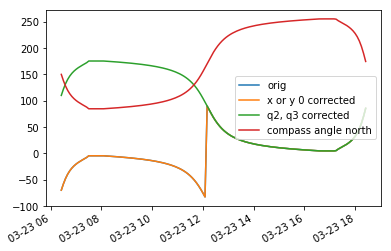The final surface_azimuth is given by the curve labeled "compass angle north". This is in degrees East of North.

#### Surface tilt¶

Calculate surface_tilt.

In :
surface_tilt = (90 - np.degrees(np.arccos(
pd.DataFrame(panel_norm_earth * projected_normal, index=times).sum(axis=1))))

surface_tilt.plot()

Out:
<matplotlib.axes._subplots.AxesSubplot at 0x1121cd668>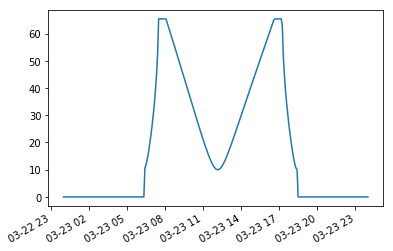According to the MATLAB code, surface_tilt is "The angle between the panel surface and the earth surface, accounting for panel rotation."

## tracking.singleaxis examples¶

With backtracking

In :
tracker_data = pvlib.tracking.singleaxis(ephemout['apparent_zenith'], ephemout['azimuth'],
axis_tilt=0, axis_azimuth=180, max_angle=90,
backtrack=True, gcr=2.0/7.0)

In :
tracker_data.plot()

Out:
<matplotlib.axes._subplots.AxesSubplot at 0x1123873c8>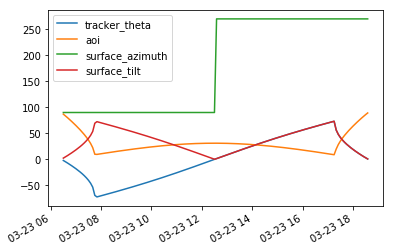Without backtracking

In :
tracker_data = pvlib.tracking.singleaxis(ephemout['apparent_zenith'], ephemout['azimuth'],
axis_tilt=0, axis_azimuth=180, max_angle=90,
backtrack=False, gcr=2.0/7.0)
tracker_data.plot()

Out:
<matplotlib.axes._subplots.AxesSubplot at 0x112530390>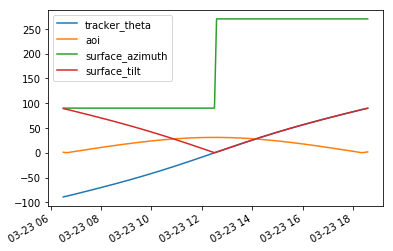Explore ground cover ratio

In :
aois = pd.DataFrame(index=ephemout.index)

for gcr in np.linspace(0, 1, 6):
tracker_data = pvlib.tracking.singleaxis(ephemout['apparent_zenith'], ephemout['azimuth'],
axis_tilt=0, axis_azimuth=180, max_angle=90,
backtrack=True, gcr=gcr)
aois[gcr] = tracker_data['aoi']

/Users/holmgren/git_repos/pvlib2/pvlib-python/pvlib/tracking.py:409: RuntimeWarning: divide by zero encountered in double_scalars
axes_distance = 1/gcr

In :
aois.plot()

Out:
<matplotlib.axes._subplots.AxesSubplot at 0x1126516a0>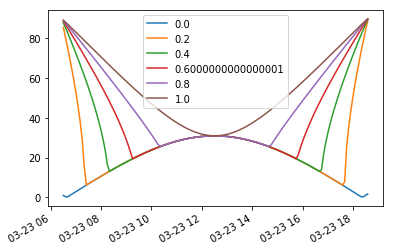Ensure that max_angle works.

In :
tracker_data = pvlib.tracking.singleaxis(ephemout['apparent_zenith'], ephemout['azimuth'],
axis_tilt=0, axis_azimuth=180, max_angle=45,
backtrack=True, gcr=2.0/7.0)
tracker_data.plot()

Out:
<matplotlib.axes._subplots.AxesSubplot at 0x111eecc50>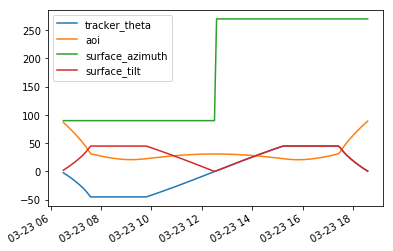Play with axis_tilt.

In :
aois = pd.DataFrame(index=ephemout.index)
tilts = pd.DataFrame(index=ephemout.index)
azis = pd.DataFrame(index=ephemout.index)
thetas = pd.DataFrame(index=ephemout.index)

for tilt in np.linspace(0, 90, 7):
tracker_data = pvlib.tracking.singleaxis(ephemout['apparent_zenith'], ephemout['azimuth'],
axis_tilt=tilt, axis_azimuth=180, max_angle=90,
backtrack=True, gcr=2/7.)
aois[tilt] = tracker_data['aoi']
tilts[tilt] = tracker_data['surface_tilt']
azis[tilt] = tracker_data['surface_azimuth']
thetas[tilt] = tracker_data['tracker_theta']

fig, axes = plt.subplots(2, 2, figsize=(16,12), sharex=True)
ax = axes[0,0]
aois.plot(ax=ax)
ax.set_ylim(0,90)
ax.set_title('aoi')

ax = axes[0,1]
thetas.plot(ax=ax)
ax.set_ylim(-90,90)
ax.set_title('tracker theta')

ax = axes[1,1]
tilts.plot(ax=ax)
ax.set_title('surface tilt')
ax.set_ylim(0,90)

ax = axes[1,0]
azis.plot(ax=ax)
ax.set_title('surface azimuth')
ax.set_ylim(0,360)
#ax.hlines([0, 90, 180, 270, 360], *ax.get_xlim(), colors='0.25', lw=1, alpha=0.25)

/Users/holmgren/git_repos/pvlib2/pvlib-python/pvlib/tracking.py:542: RuntimeWarning: invalid value encountered in arccos
index=times).sum(axis=1))))

Out:
(0, 360)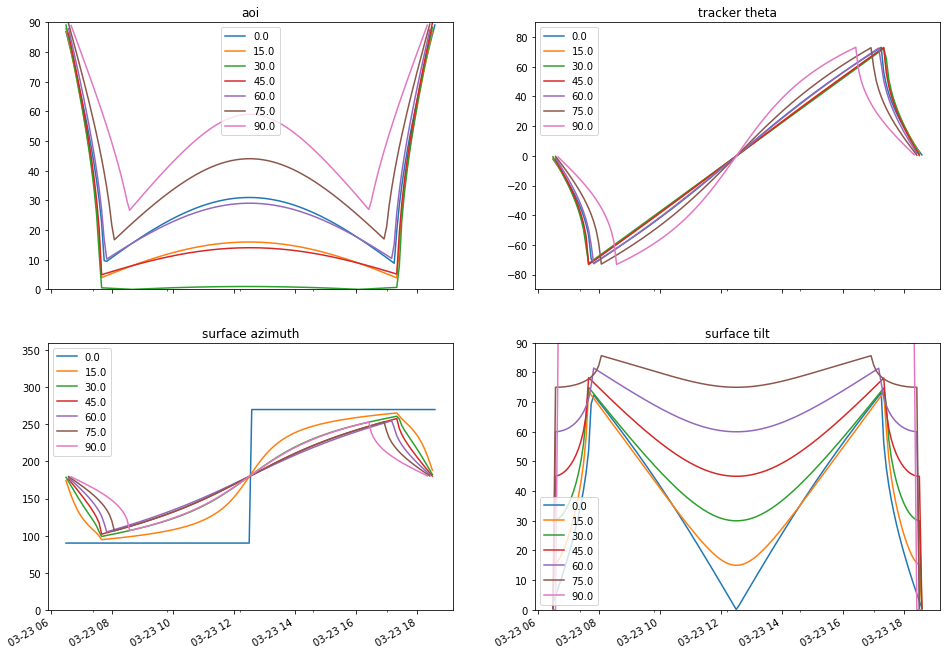The simple case of axis_tilt = 0 shows the panels pointing directly East in the morning and directly West in the afternoon. If axis_tilt > 0 then the panels always point South of East and South of West. The panels point towards South near sunrise, rotate towards East in mid-morning, then back towards Sorth around noon, continuing towards West in the mid-afternoon, and finally back towards Sorth near sunset.

Next, what happens if we try to point the panels North?

In :
aois = pd.DataFrame(index=ephemout.index)
tilts = pd.DataFrame(index=ephemout.index)
azis = pd.DataFrame(index=ephemout.index)
thetas = pd.DataFrame(index=ephemout.index)

for tilt in np.linspace(0, -90, 7):
tracker_data = pvlib.tracking.singleaxis(ephemout['apparent_zenith'], ephemout['azimuth'],
axis_tilt=tilt, axis_azimuth=180, max_angle=90,
backtrack=True, gcr=2/7.)
aois[tilt] = tracker_data['aoi']
tilts[tilt] = tracker_data['surface_tilt']
azis[tilt] = tracker_data['surface_azimuth']
thetas[tilt] = tracker_data['tracker_theta']

fig, axes = plt.subplots(2, 2, figsize=(16,12), sharex=True)
ax = axes[0,0]
aois.plot(ax=ax)
ax.set_ylim(0,90)
ax.set_title('aoi')

ax = axes[0,1]
thetas.plot(ax=ax)
ax.set_ylim(-90,90)
ax.set_title('tracker theta')

ax = axes[1,1]
tilts.plot(ax=ax)
ax.set_title('surface tilt')
ax.set_ylim(0,90)

ax = axes[1,0]
azis.plot(ax=ax)
ax.set_title('surface azimuth')
ax.set_ylim(0,360)
#ax.hlines([0, 90, 180, 270, 360], *ax.get_xlim(), colors='0.25', lw=1, alpha=0.25)

/Users/holmgren/git_repos/pvlib2/pvlib-python/pvlib/tracking.py:542: RuntimeWarning: invalid value encountered in arccos
index=times).sum(axis=1))))

Out:
(0, 360)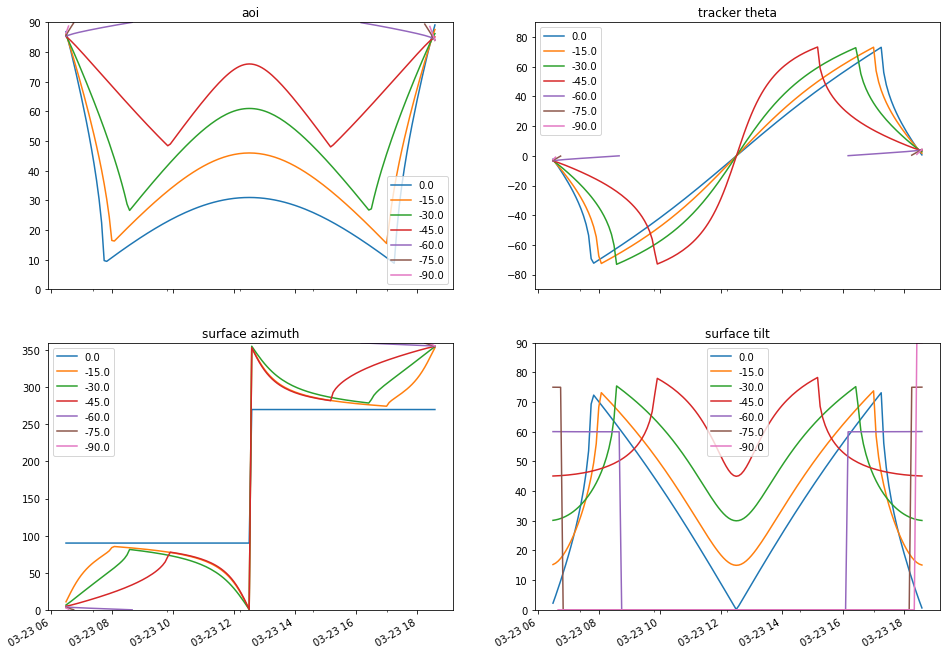The 0 tilt case is repeated for reference. For small Northward tilts, such as -15, the panels point towards North near sunrise, rotate towards the east in mid-morning, then back towards North around noon, continuing towards West in the mid-afternoon, and finally back towards North near sunset.

The algorithm returns nan for larger Northward tilts at midday since the beam component of the irradiance would be 0.

Play with axis_azimuth.

In :
aois = pd.DataFrame(index=ephemout.index)
tilts = pd.DataFrame(index=ephemout.index)
azis = pd.DataFrame(index=ephemout.index)
thetas = pd.DataFrame(index=ephemout.index)

for azi in np.linspace(90, 270, 5):
tracker_data = pvlib.tracking.singleaxis(ephemout['apparent_zenith'], ephemout['azimuth'],
axis_tilt=0, axis_azimuth=azi, max_angle=90,
backtrack=True, gcr=2/7.)
aois[azi] = tracker_data['aoi']
tilts[azi] = tracker_data['surface_tilt']
azis[azi] = tracker_data['surface_azimuth']
thetas[azi] = tracker_data['tracker_theta&#